Related Articles

# Change data type of given numpy array

• Difficulty Level : Easy
• Last Updated : 23 Jun, 2021

In this post, we are going to see the ways in which we can change the dtype of the given numpy array. In order to change the dtype of the given array object, we will use `numpy.astype()` function. The function takes an argument which is the target data type. The function supports all the generic types and built-in types of data.

Problem #1 : Given a numpy array whose underlying data is of `'int32'` type. Change the dtype of the given object to `'float64'`.

Solution : We will use `numpy.astype()` function to change the data type of the underlying data of the given numpy array.

 `# importing the numpy library as np``import` `numpy as np`` ` `# Create a numpy array``arr ``=` `np.array([``10``, ``20``, ``30``, ``40``, ``50``])`` ` `# Print the array``print``(arr)`

Output :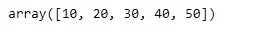Now we will check the dtype of the given array object.

 `# Print the dtype``print``(arr.dtype)`

Output :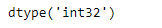As we can see in the output, the current dtype of the given array object is ‘int32’. Now we will change this to ‘float64’ type.

 `# change the dtype to 'float64'``arr ``=` `arr.astype(``'float64'``)`` ` `# Print the array after changing``# the data type``print``(arr)`` ` `# Also print the data type``print``(arr.dtype)`

Output :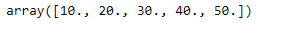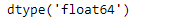Problem #2 : Given a numpy array whose underlying data is of `'int32'` type. Change the dtype of the given object to `'complex128'`.

Solution : We will use `numpy.astype()` function to change the data type of the underlying data of the given numpy array.

 `# importing the numpy library as np``import` `numpy as np`` ` `# Create a numpy array``arr ``=` `np.array([``10``, ``20``, ``30``, ``40``, ``50``])`` ` `# Print the array``print``(arr)`

Output :Now we will check the dtype of the given array object.

 `# Print the dtype``print``(arr.dtype)`

Output :As we can see in the output, the current dtype of the given array object is ‘int32’. Now we will change this to ‘complex128’ type.

 `# change the dtype to 'complex128'``arr ``=` `arr ``=` `arr.astype(``'complex128'``)`` ` `# Print the array after changing``# the data type``print``(arr)`` ` `# Also print the data type``print``(arr.dtype)`

Output :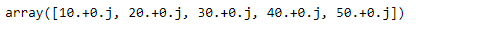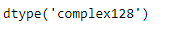Attention geek! Strengthen your foundations with the Python Programming Foundation Course and learn the basics.

To begin with, your interview preparations Enhance your Data Structures concepts with the Python DS Course. And to begin with your Machine Learning Journey, join the Machine Learning – Basic Level Course

My Personal Notes arrow_drop_up